×#### Thank you for registering.

One of our academic counsellors will contact you within 1 working day.

Click to Chat

1800-1023-196

+91-120-4616500

CART 0

• 0

MY CART (5)

Use Coupon: CART20 and get 20% off on all online Study Material

ITEM
DETAILS
MRP
DISCOUNT
FINAL PRICE
Total Price: Rs.

There are no items in this cart.
Continue Shopping• Complete JEE Main/Advanced Course and Test Series
• OFFERED PRICE: Rs. 15,900
• View Details

```Revision Notes on Perimeter and Area

Perimeter

It refers to the length of the outline of the enclosed figure.

Area

It refers to the surface of the enclosed figure.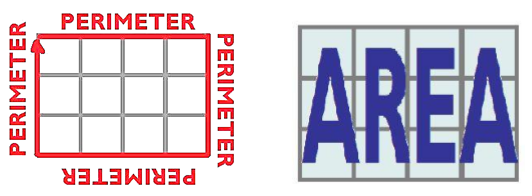Area and Perimeter of Square

Square is a quadrilateral, with four equal sides.

Area = Side × Side

Perimeter = 4 × Side

Example

Find the area and perimeter of a square-shaped cardboard whose length is 5 cm.Solution

Area of square = (side)2

= (5)2

= 25 cm2

Perimeter of square = 4 × side

= 4 × 5

= 20 cm

Area and Perimeter of Rectangle

The rectangle is a quadrilateral, with equal opposite sides.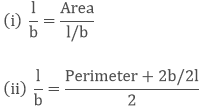Example

What is the length of a rectangular field if its width is 20 ft and Area is 500 ft2?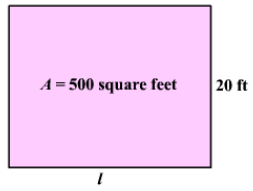Solution

Area of rectangular field = length × width

500 = l × 20

l = 500/20

l = 25 ft

Note: Perimeter of a regular polygon = Number of sides × length of one side

Triangles as Parts of Rectangles

If we draw a diagonal of a rectangle then we get two equal sizes of triangles. So the area of these triangles will be half of the area of a rectangle.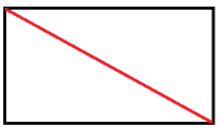The area of each triangle = 1/2 (Area of the rectangle)

Likewise, if we draw two diagonals of a square then we get four equal sizes of triangles .so the area of each triangle will be one-fourth of the area of the square.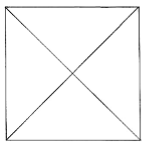The area of each triangle = 1/4 (Area of the square)

Example

What will be the area of each triangle if we draw two diagonals of a square with side 7 cm?

Solution

Area of square = 7 × 7

= 49 cm2

The area of each triangle = 1/4 (Area of the square)

= 1/4 × 49

= 12.25 cm2

Congruent Parts of Rectangles

Two parts of a rectangle are congruent to each other if the area of the first part is equal to the area of the second part.

Example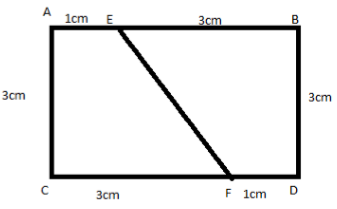The area of each congruent part = 1/2 (Area of the rectangle)

= 1/2 (l × b) cm2

=1/2 (4 × 3) cm2

= 1/2 (12) cm2

= 6 cm2

Parallelogram

It is a simple quadrilateral with two pairs of parallel sides.

Also denoted as ∥ gm

Area of parallelogram = base × height

Or b × h (bh)

We can take any of the sides as the base of the parallelogram. And the perpendicular drawn on that side from the opposite vertex is the height of the parallelogram.

Example

Find the area of the figure given below:Solution

Base of ∥ gm = 8 cm

Height of ∥ gm = 6 cm

Area of ∥ gm = b × h

= 8 × 6

= 48 cm

Area of Triangle

Triangle is a three-sided closed polygon.

If we join two congruent triangles together then we get a parallelogram. So the area of the triangle will be half of the area of the parallelogram.

Area of Triangle = 1/2 (Area of ∥ gm)

= 1/2 (base × height)

Example

Find the area of the figure given below: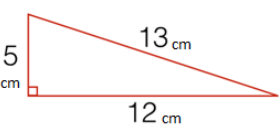Solution

Area of triangle = 1/2 (base × height)

= 1/2 (12 × 5)

= 1/2 × 60

= 30 cm2

Note: All the congruent triangles are equal in area but the triangles equal in the area need not be congruent.

Circles

It is a round, closed shape.

The circumference of a Circle

The circumference of a circle refers to the distance around the circle.

Radius: A straight line from the Circumference till the centre of the circle.

Diameter: It refers to the line from one point of the Circumference to the other point of the Circumference.

π (pi): It refers to the ratio of a circle's circumference to its diameter.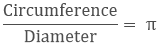Circumference(c) = π × diameter

C = πd

= π × 2r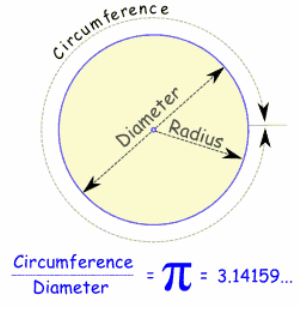Note: diameter (d) = twice the radius (r)

d = 2r

Example

What is the circumference of a circle of diameter 12 cm (Take π = 3.14)?

Solution

C = πd

C = 3.14 × 12

= 37.68 cm

Area of Circle

Area of the circle = (Half of the circumference) × radius

= πr2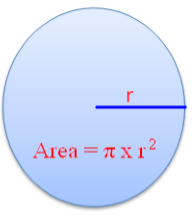Example

Find the area of a circle of radius 23 cm (use π = 3.14).

Solution

R = 23 cm

π = 3.14

Area of circle = 3.14 × 232

= 1,661 cm2

Conversion of Units

Sometimes we need to convert the unit of the given measurements to make it similar to the other given units.

Unit
Conversion

1 cm
10 mm

1 m
100 cm

1 km
1000 m

1 hectare(ha)
100 × 100 m

Unit
Conversion

1 cm2
100 mm2

1 m2
10000 cm2

1 km2
1000000 m2 (1e + 6)

1 ha
10000 m2

Example: 1

Convert 70 cm2 in mm2

Solution:

1 cm = 10 mm

1 cm2 = 10 × 10

1 cm2 = 100 mm2

70 cm2 = 700 mm2

Example: 2

Convert 3.5 ha in m2

Solution:

1 ha = 10000 m2

3.5 ha = 10000 × 3.5

ha = 35000 m2

Applications

We can use these concepts of area and perimeter of plane figures in our day to day life.

If we have a rectangular field and want to calculate that how long will be the length of the fence required to cover that field, then we will use the perimeter.

If a child has to decorate a circular card with the lace then he can calculate the length of the lace required by calculating the circumference of the card, etc.

Example:

A rectangular park is 35 m long and 20 m wide. A path 1.5 m wide is constructed outside the park. Find the area of the path.Solution

Area of rectangle ABCD – Area of rectangle STUV

AB = 35 + 2.5 + 2.5

= 40 m

AD = 20 + 2.5 + 2.5

= 25 m

Area of ABCD = 40 × 25

= 1000 m2

Area of STUV = 35 × 20

= 700 m2

Area of path = Area of rectangle ABCD – Area of rectangle STUV

= 1000 – 700

= 300 m2
```### Course Features

• 728 Video Lectures
• Revision Notes
• Previous Year Papers
• Mind Map
• Study Planner
• NCERT Solutions
• Discussion Forum
• Test paper with Video Solution